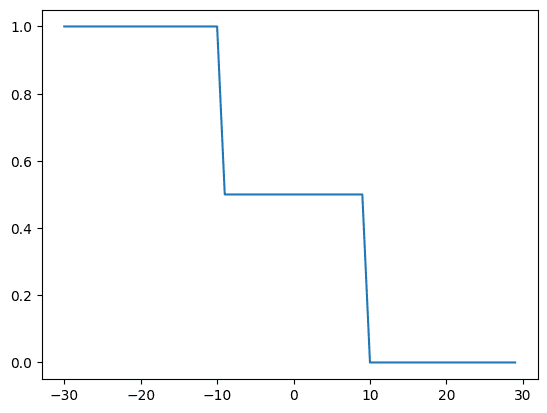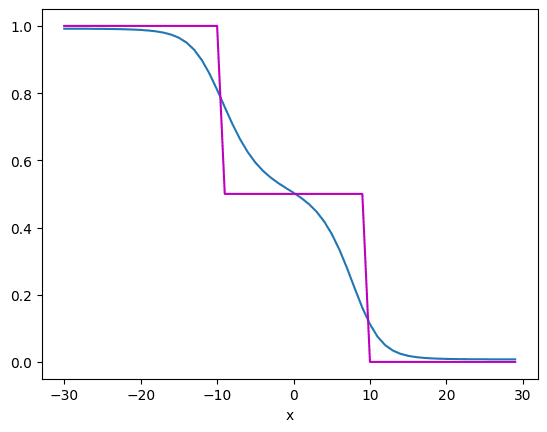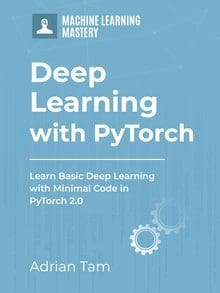# Building a Single Layer Neural Network in PyTorch

A neural network is a set of neuron nodes that are interconnected with one another. The neurons are not just connected to their adjacent neurons but also to the ones that are farther away.

The main idea behind neural networks is that every neuron in a layer has one or more input values, and they produce output values by applying some mathematical functions to the input. The outputs of the neurons in one layer become the inputs for the next layer.

A single layer neural network is a type of artificial neural network where there is only one hidden layer between the input and output layers. This is the classic architecture before the deep learning became popular. In this tutorial, you will get a chance to build a neural network with only a single hidden layer. Particularly, you will learn:

• How to build a single layer neural network in PyTorch.
• How to train a single layer neural network with PyTorch.
• How to classify one-dimensional data using a single layer neural network.

Kick-start your project with my book Deep Learning with PyTorch. It provides self-study tutorials with working code.

Let’s get started.Building a Single Layer Neural Network in PyTorch.
Picture by Tim Cheung. Some rights reserved.

## Overview

This tutorial is in three parts; they are

• Preparing the Dataset
• Build the Model
• Train the Model

## Preparing the Data

A neural network simply a function that approximates other functions with some parameters. Let’s build some data and see how our single layer neural network approximates the function to make the data linearly separable. Later in this tutorial, you will visualize the function during training to see how the approximated function overlaps over the given set of data points.

The data, as plotted using matplotlib, looks like the following.### Want to Get Started With Deep Learning with PyTorch?

Take my free email crash course now (with sample code).

Click to sign-up and also get a free PDF Ebook version of the course.

## Build the Model with nn.Module

Next, let’s build our custom module for single layer neural network with nn.Module. Please check previous tutorials of the series if you need more information on nn.Module.

This neural network features an input layer, a hidden layer with two neurons, and an output layer. After each layer, a sigmoid activation function is applied. Other kind of activation functions are available in PyTorch but the classic design for this network is to use sigmoid function.

Here is how your single layer neural network looks like in code.

Let’s also instantiate a model object.

## Train the Model

Before starting the training loop, let’s define loss function and optimizer for the model. You will write a loss function for the cross entropy loss and use stochastic gradient descent for parameter optimization.

Now you have all components to train the model. Let’s train the model for 5000 epochs. You will see a plot of how the neural network approximates the function after every 1000 epochs.

After 1000 epochs, the model approximated the function like the following:But after 5000 epochs, it improves to the following:From which, you can see the approximation in blue is closer to the data in purple. As you can see, the neural network approximates the functions quite nicely. If the function is more complex, you may need more hidden layers or more neurons in the hidden layer, i.e., a more complex model.

Let’s also plot to see how the loss reduced during training.

You should see:Putting everything together, the following is the complete code:

## Summary

In this tutorial, you learned how you can build and train a neural network and estimate the function. Particularly, you learned:

• How to build a single layer neural network in PyTorch.
• How to train a single layer neural network with PyTorch.
• How to classify one-dimensional data using a single layer neural network.

## Get Started on Deep Learning with PyTorch!#### Learn how to build deep learning models

...using the newly released PyTorch 2.0 library

Discover how in my new Ebook:
Deep Learning with PyTorch

It provides self-study tutorials with hundreds of working code to turn you from a novice to expert. It equips you with
tensor operation, training, evaluation, hyperparameter optimization, and much more...

### 3 Responses to Building a Single Layer Neural Network in PyTorch

1.Anthony the Koala January 14, 2023 at 10:57 am #

I have followed Mr Khan’s tutorials.

First, they are clearly written and secondly a by-product of his tutorials are the application of instatiating classes and inheritance from superior classes and implementaion of inherited classes.

Thank you,
Anthony of Sydney

2.Alex.A January 14, 2023 at 9:40 pm #

Hi, followed the code, using jupyter notebook, works well.

The epochs calculations takes time. How can I convert the code to run with CUDA, to accelarate the speed?